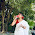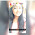## Wednesday, 22 August 2012

### CBSE - Class 9 - Maths - Lines and Angles (Ex. 6.3)

Lines and Angles
(NCERT Ex. 6.3 + Q & A from CBSE papers)

Points to remember
1. The sum of the angles of a triangle is 180º.Impossible Triangle (credits:openclipart)
2. If a side of a triangle is produced, then the exterior angle so formed is equal to the sum of the two interior opposite angles.

3. An exterior angle of a triangle is greater than either of its interior opposite angles.

4. If one angle of a triangle is a right angle, then the other two angles are acute angles. (Thus no triangle can have two right angles.)

5. The two acute angles in every right triangle are complementary.

6. The sum of all interior angles of an n-sided polygon is (n -2) × 180°

7. The sum of all exterior angles of an n-sided polygon is 360°.(one exterior angle at each vertex)

Exercise 6.3

Q1: In Fig. below, sides QP and RQ of ΔPQR are produced to points S and T respectively.
If ∠SPR = 135° and ∠PQT = 110°, find ∠PRQ.Given, ∠SPR = 135° and ∠PQT = 110°
∵ ∠SPR and ∠QPR are linear pair.
∠SPR + ∠QPR = 180°
⇒  ∠QPR = 180° - ∠SPR = 180° - 135° = 45°

∠PQT is an exterior angle of ΔPQR and is equal to sum of its interior angles.
⇒  ∠PQT = ∠QPR + ∠PRQ
⇒ 110° = 45° + ∠PRQ
⇒ ∠PRQ = 110° - 45° = 65°

Q2: In Fig. below, ∠X = 62°, ∠XYZ = 54°. If YO and ZO are the bisectors of ∠XYZ and ∠XZY respectively of ΔXYZ, find ∠OZY and ∠YOZ.

Answer: Given ∠X = 62°, ∠XYZ = 54°
Since the sum of the angles of a triangle is 180º.
⇒  ∠X +  ∠XYZ + ∠XZY = 180°
⇒ ∠XZY = 180° - (∠X +  ∠XYZ) = 180° - (62° + 54°)
⇒ ∠XZY  = 180° - 116° = 64°

Since YO is bisector of  ∠XYZ
⇒ ∠OYZ = ∠XYZ/2 = 54°/2 = 27°
Similarly,   ZO is bisector of ∠XZY
⇒ ∠OZY = ⇒ ∠XZY/2 = 64°/2 = 32°

Using angle sum property of a ΔOYZ,
∠OYZ + ∠O + ∠OZY = 180°
⇒ ∠O  = 180° - (∠OYZ + ∠OZY) = 180° - (27° + 32°) = 180° - 59° = 121°

Q3: In Fig. below, if AB || DE, ∠ BAC = 35° and ∠ CDE = 53°, find ∠ DCE.

Answer:  Since AB || DE and AE is transversal.
⇒ ∠BAC = ∠CED       (Alternate interior angles)
⇒ ∠CED = 35°

In  ΔCDE, using angle sum property of a triangle.
∠CDE + ∠DCE + ∠CED = 180°
⇒  53° + ∠DCE + 35° = 180°
⇒ ∠DCE = 180° - 88° = 92°

Q4: In Figure below, if lines PQ and RS intersect at point T, such that ∠PRT = 40°, ∠RPT = 95° and ∠TSQ = 75°, find ∠SQT.

Answer:  Using angle sum property in ΔPRT,
∠PRT + ∠RPT + ∠PTR = 180°
⇒ ∠PTR = 180° - (∠PRT + ∠RPT)
⇒ ∠PTR = 180° - (40° + 95°) = 180° - 135°
∠PTR = 45°

∠PTR and ∠STQ are vertically opposite angles and are equal.
∴ ∠STQ = ∠PTR = 45°

Using angle sum property in  ΔSTQ.
⇒ ∠STQ + ∠TSQ + ∠SQT = 180°
⇒ ∠SQT = 180° - (∠STQ + ∠TSQ) = 180° - (45° + 75°) = 180° - 120° = 60°

Q5: In Fig. shown below, if PQ ⊥ PS, PQ || SR, ∠SQR = 28° and ∠QRT = 65°, then find the values of x and y.

Answer: Since PQ || SR and QR is transversal   (Given),
∠QRT = ∠PQR   (alternate interior angle)
⇒ ∠PQR = 65°

Since  ∠PQR = x + 28°

⇒ x + 28° = 65°
⇒ x = 65° - 28° = 37°

Using angle sum property in ΔPQS,
x + y + ∠SPQ = 180°
y = 180° - (∠SPQ + x) = 180° - (90° + 37°)                                      (∵ PQ ⊥ PS)
⇒ y = 180° - 127° = 53°

Q6: In Fig. below, the side QR of Δ PQR is produced to a point S. If the bisectors of ∠PQR and ∠PRS meet at point T, then prove that ∠QTR = ½ ∠QPR.

Answer:  The above figure consists of two overlapped triangles i.e. ΔPQR and ΔQRT.

In ΔQRT, ∠TRS is an exterior angle.
⇒ ∠TRS = ∠QTR + ∠TQR
⇒ ∠QTR =  ∠TRS - ∠TQR                ....(I)

Similarly, in ΔPQR, ∠PRS is an external angle
⇒ ∠PRS = ∠PQR + ∠QPR
⇒ 2∠TRS = 2∠TQR + ∠QPR         (∵ QT and TR are bisectors of  ∠PQR and ∠PRS)
⇒ 2 (∠TRS - ∠TQR) = ∠QPR

From eqn. (I) we have,
2 ( ∠QTR) = ∠QPR
⇒ ∠QTR = ½ ∠QPR.

Q7: Can a triangle have:
(a) two right angles?
(b) two obtuse angles?
(c) two acute angles?
(d) all angles less than 60°
(e) all angles more than 60°
(f)  all angles equal to 60°

(a)  No. Since the sum of three angles of a triangle is 180°. Sum of two right angles is 180, such triangle is not possible.

(b) No. Because the sum of two obtuse angles will become greater than 180°. Such a triangle is not feasible.

(c) Yes a triangle can have two acute angles. Since the sum of two acute angle is less than 180°, the third angle will have the remaining value.

(d) Since sum of all angles less than 60° is still less than 180°. Such a triangle is not possible.

(e) Since sum of angles (each angle is greater than 60°) exceed 180°, such a triangle is not possible.

(f) Sum of angles (each = 60°) is exactly equal to 180°, such a triangle is possible. It makes an equilateral triangle.

Q8: How many exterior angles does a triangle have?

Answer: Six. At a vertex of a triangle, there are two exterior angles. A triangle has three vertices, thus it has six exterior angles.

Q9: In a ΔABC, A° = 2B° and  = 3C°. Find the values of all the angles of the Δ.

Answer:  Using angle sum property of the triangle,
A° + B° + C° = 180°
⇒  2B° + B° + C° = 180°                  (Given, A° = 2B°)
⇒  3B° + C° = 180°
⇒ 3(3C°) + C° = 180°                       (Given B° = 3C°)
⇒ 9C° + C° = 180°
⇒ 10C° = 180°
⇒ C° = 180°/10 = 18°
∴ B = 3 × 18° = 54°
and A = 2  × 54° = 108°

Q10: The angles of a triangle are in the ratio 5 : 3 : 7. The triangle is :

(a) an acute angled triangle
(b) an obtuse angled triangle
(c) a right triangle
(d) an isosceles triangle

Answer: (a) an acute angled triangle
Let the angles be 5x, 3x and 7x. Using angle sum property, 5x + 3x + 7x = 180°
⇒ 15x = 180°
⇒ x = 12°
∴ Angles of the Δ are: 60°, 36°, 84° - an acute angle triangle.Q11(CBSE 2010):In the figure, if x°, y° and z° are exterior angles of Δ ABC, then x° + y° + z° is:
(a) 180°
(b) 360°
(c) 270°
(d) 90°

In simple polygons, the sum exterior angles (one exterior angle at each vertex) is always 360°.

Q12(CBSE 2010): In the given figure, the measure of ∠ABC is:

(a) 80°
(b) 20°
(c) 100°
(d) 60°

Q13: An exterior angle of a triangle is 130° and its two interior opposite angles are equal. Each of the interior angle is equal to:

(a) 45°
(b) 65°
(c) 75°
(d) 35°1.whats the reason of the last answer??...

1.Q12: ∠BAC = 20° (vertically opp. angle)
∠ABC + ∠BAC = 100° (exterior angle of Δ is sum of opposite interior angles) ⇒∠ABC = 80°

2.a small spelling mistake in the 2nd point of POINTS TO REMEMBER its written apposite instead of opposite

3.sorry not the 2nd one the 3rd one

4.there is even numbering mistake pls do correct

1.Both points are fixed. Thanks for reviewing it. It helps in improving the blog.

5.it was nice but i was not understand Q9.

1.Hmmmm....its quiet tough

2.Hmmmm....its quiet tough

6.Good questions❤❤
I enjoyed doing all these😘

7.if the angles of a triangle is in ratio 5:3:7

form than show that the triangle is an accute angled triangle

8.if the angles of a triangle is in ratio 5:3:7

form than show that the triangle is an accute angled triangle

1.Take all the angles x 5x+3x+7x equal to 180.. then find the value of x and then multiple it with 5,3 and 7 that's it

2.Take all the angles x 5x+3x+7x equal to 180.. then find the value of x and then multiple it with 5,3 and 7 that's it

9.All are easy .... Just solve RS Aggarwal once, and you can easily solve all of the question with on mistake ....

10.All are easy .... Just solve RS Aggarwal once, and you can easily solve all of the question with on mistake ....

11.Thank you very much .....it was very helpfull

12.Buy you must visit my blog computer--knowledge.blogspot.com

13.I cant understand number 12